The ForeCA R package implements forecastable compoenent analysis (ForeCA).

library(ForeCA)


If you don't know about ForeCA yet, the next section gives a quick overview. If you know ForeCA already you can skip it and go straight to the examples.

## What is ForeCA?

Forecastable component analysis (ForeCA) is a novel dimension reduction (DR) technique for multivariate time series. Similar to other DR methods ForeCA tries to find an “interesting” linear combination $$y_t = \mathbf{w}‘ X_t$$ of a multivariate time series $$\mathbf{X}_t$$. For principal component analysis (PCA) high variance data is interesting; for slow feature analysis (SFA) slow signals are interesting. ForeCA tries to find linear combinations that are easy to forecast, i.e., forecastable – hence the name.

The measure of forecastability, denoted as $$\Omega(y_t)$$, is based on the spectral entropy of $$y_t$$, i.e., the entropy of the spectral density $$f_y(\lambda)$$: high entropy means low forecastability; low entropy signals are easy to forecast.

For more details see the original ForeCA paper:

citation("ForeCA")

##
## If you use 'ForeCA' in your publication we ask you to cite both the
## 'ForeCA' R package as well as the original ForeCA article in JMLR:
##
##   Georg M. Goerg (2020). ForeCA: An R package for Forecastable
##   Component Analysis. R package version 0.2.7.
##
##   Georg M. Goerg: Forecastable Component Analysis. JMLR, W&CP (2) 2013:
##   64-72.
##
## To see these entries in BibTeX format, use 'print(<citation>,
## bibtex=TRUE)', 'toBibtex(.)', or set
## 'options(citation.bibtex.max=999)'.


# Specifying estimation settings

ForeCA uses two main estimation techniques:

• spectral density estimation of multivariate (or univariate) time series $$\mathbf{X}_t$$ (or $$y_t$$);
• Shannon entropy estimation of a univariate probability mass function.

The ForeCA package achieves this via

• astsa package for good non-parametric estimates of the spectrum, and
• simple plugin estimates of Shannon entropy (discrete_entropy / continuous_entropy) with optional prior smoothing.

In this package details of those two estimator are specified via spectrum.control and entropy.control arguments (lists). For sake of simplicity let's fix them here and use those settings all throughout the examples.

# spectrum control
sc <- list(method = "mvspec")
# entropy control
ec <- list(prior.weight = 1e-2)


For more options and detailed explanations see ?complete-controls.

# Example: European stock market data

data("EuStockMarkets")


The EuStockMarkets data contains daily closing prices for 4 major European stock markets. As usual we convert this to log-returns to make the time series stationary.

# log-returns in %
ret <- diff(log(EuStockMarkets)) * 100


## Time series EDA

ret contains time series of 4 major European stock indices (see ?EuStockMarkets for details).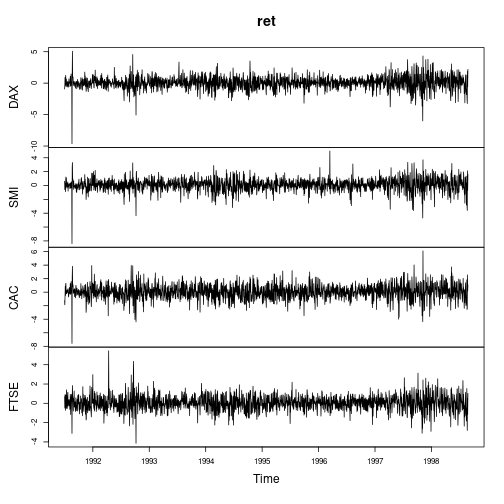cor.ret <- cor(ret)
kable(format(cor.ret, digits = 2),
caption = "Correlation matrix")


Table: Correlation matrix

DAX SMI CAC FTSE
DAX 1.00 0.70 0.73 0.64
SMI 0.70 1.00 0.62 0.58
CAC 0.73 0.62 1.00 0.65
FTSE 0.64 0.58 0.65 1.00
kable(format(solve(cor.ret), digits = 2),
caption = "Conditional covariance given other variables")


Table: Conditional covariance given other variables

DAX SMI CAC FTSE
DAX 2.90 -1.03 -1.18 -0.49
SMI -1.03 2.14 -0.31 -0.40
CAC -1.18 -0.31 2.51 -0.69
FTSE -0.49 -0.40 -0.69 1.99

The correlation matrix shows that they are highly correlated with each other and the partial autocorrelation function (PACF) and spectra show that they are slightly autocorrelated.

ret.spec <- mvspectrum(ret, method = sc$method) plot(ret.spec)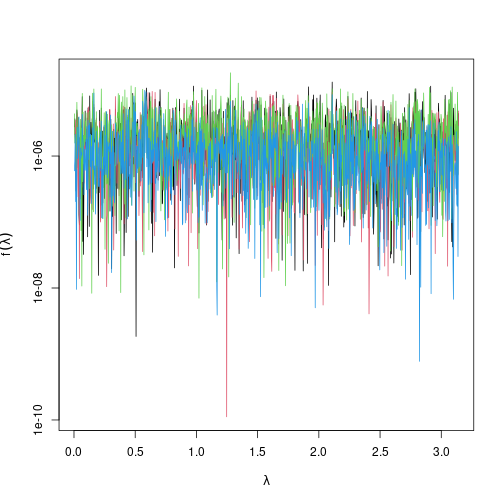layout(matrix(seq_len(ncol(ret)), ncol = 2)) for (nn in colnames(ret)) { pacf(ret[, nn], main = nn) }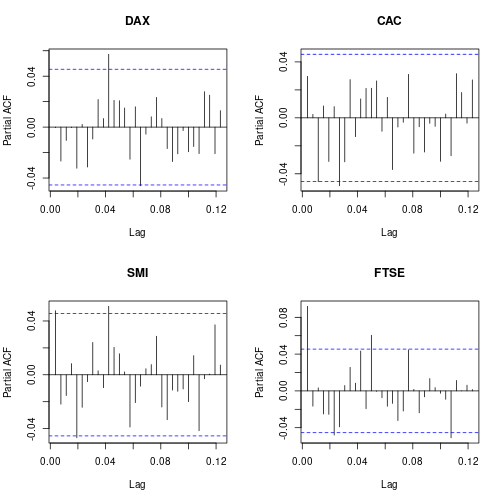Not surprisingly the PACF shows only very small partial correlations, since these are stock market return and we should not expect see too large correlations over time (cf. “no arbitrage” hypothesis). More specifically, we can estimate ForeCA measure of forecastability, $$\Omega$$, for each series: ret.omega <- Omega(ret, spectrum.control = sc, entropy.control = ec) ret.omega  ## DAX SMI CAC FTSE ## 5.353323 5.135365 4.966076 5.253096 ## attr(,"unit") ##  "%"  According to the estimates CAC is the least forecastable, DAX is the most forecastable stock market. However, we can ask if there are linear combinations of stock markets, i.e., a portfolio, that are even easier to forecast. That's exactly what ForeCA is doing. ## foreca(): find forecastable components The main function in the package is foreca(), which should be straightforward to use as it resembles princomp for PCA or fastICA for independent component anlaysis (ICA). In the basic setting users only have to pass the multivariate time series and the number of components (n.comp – by default it uses 2). We also specify spectrum.control and entropy.control but this is optional. mod.foreca.ret <- foreca(ret, n.comp = ncol(ret), spectrum.control = sc, entropy.control = ec)  mod.foreca.ret # this uses the print.foreca method  ## ForeCA found the top 4 ForeCs of 'ret' (4 time series). ## Out of the top 4 ForeCs, 1 are white noise. ## ## Omega(ForeC 1) = 6.2% vs. maximum Omega(ret) = 5.35%. ## This is an absolute increase of 0.85 percentage points (relative: 15.89%) in forecastability. ## ## * * * * * * * * * * ## Use plot(), biplot(), and summary() for more details.  For ease of use and better integration with existing methods in R, the returned foreca objects are very similar to princomp objects, i.e., they have $scores and $loadings (and many other useful metrics). str(mod.foreca.ret) # too much to display; try it out!  The console print out showed that ForeCA indeed worked and it could find linear combinations that were more forecastable than any of the original series. The $$\Omega$$ score of the forecastable components (ForeCs) are mod.foreca.ret$Omega

##   ForeC1   ForeC2   ForeC3   ForeC4
## 6.203952 6.073840 5.729620 5.059563


Recall that the most forecastable original time series had $$\hat{\Omega} = 5.35$$ (use summary(mod.foreca.ret)$Omega.orig) to get them). The loadings of the ForeCs tell us what this forecastable portfolio looks like: mod.foreca.ret$loadings

##
##      ForeC1 ForeC2 ForeC3 ForeC4
## DAX   1.543  0.296         0.515
## SMI  -0.891  0.718 -1.093
## CAC  -0.829  0.111  1.052  0.502
## FTSE        -1.676 -0.568
##
##                ForeC1 ForeC2 ForeC3 ForeC4
## Proportion Var  0.966  0.856  0.657  0.132
## Cumulative Var  0.966  1.822  2.479  2.611


# S3 methods

The ForeCA package implements several S3 methods for objects of class foreca so that results can be quickly visualized and easily interpreted.

plot(mod.foreca.ret)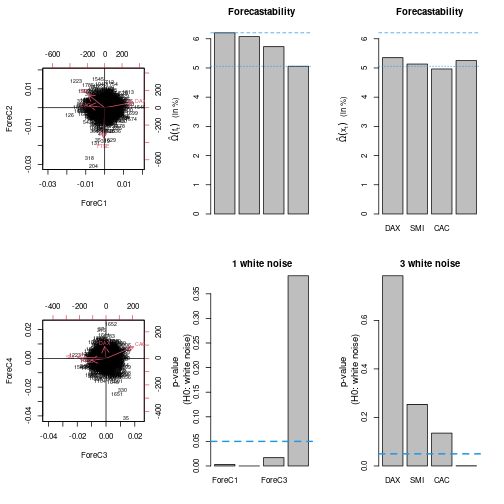plot(mod.foreca.ret$scores)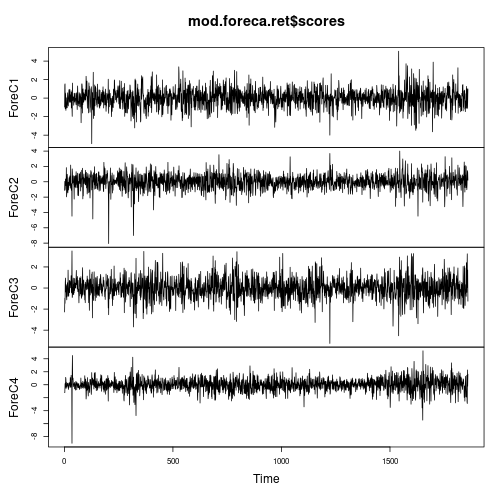By design of ForeCA, the returned series are uncorrelated and have zero mean and unit variance. round(colMeans(mod.foreca.ret$scores), 3)

## ForeC1 ForeC2 ForeC3 ForeC4
##      0      0      0      0

kable(round(cov(mod.foreca.ret\$scores), digits = 3))

ForeC1 ForeC2 ForeC3 ForeC4
ForeC1 1 0 0 0
ForeC2 0 1 0 0
ForeC3 0 0 1 0
ForeC4 0 0 0 1

# Session info

sessionInfo()

## R version 4.0.2 (2020-06-22)
## Platform: x86_64-pc-linux-gnu (64-bit)
## Running under: Ubuntu 20.04 LTS
##
## Matrix products: default
## BLAS:   /usr/lib/x86_64-linux-gnu/blas/libblas.so.3.9.0
## LAPACK: /usr/lib/x86_64-linux-gnu/lapack/liblapack.so.3.9.0
##
## locale:
##   LC_CTYPE=en_US.UTF-8       LC_NUMERIC=C
##   LC_TIME=en_US.UTF-8        LC_COLLATE=C
##   LC_MONETARY=en_US.UTF-8    LC_MESSAGES=en_US.UTF-8
##   LC_PAPER=en_US.UTF-8       LC_NAME=C
##  LC_MEASUREMENT=en_US.UTF-8 LC_IDENTIFICATION=C
##
## attached base packages:
##  stats     graphics  grDevices utils     datasets  methods   base
##
## other attached packages:
##  ForeCA_0.2.7 knitr_1.29
##
## loaded via a namespace (and not attached):
##   MASS_7.3-51.6    compiler_4.0.2   plyr_1.8.6       magrittr_1.5
##   astsa_1.10       tools_4.0.2      reshape2_1.4.4   Rcpp_1.0.4.6
##   codetools_0.2-16 stringi_1.4.6    highr_0.8        digest_0.6.25
##  stringr_1.4.0    xfun_0.15        evaluate_0.14